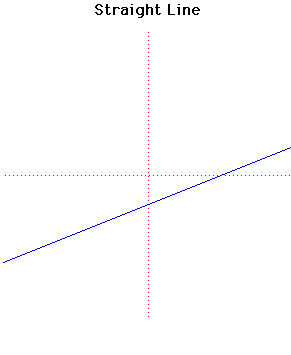# Curves

### Straight LineCartesian equation:
$y = mx + c$
or parametrically:
$x = at + b, y = ct +d$

### Description

The straight line must be one of the earliest curves studied, but Euclid in his Elementsalthough he devotes much study to the straight line, does not consider it a curve.

In fact nobody attempted a general definition of a curve until Jordan in his Cours d'Analysein 1893.

The inverse of a straight line is a circle if the centre of inversion is not on the line.

The negative pedal of the straight line is a parabola if the pedal point is not on the line.

Since normals to a straight line never intersect and tangents coincide with the curve, evolutes, involutes and pedal curves are not too interesting.

Other Web site:

Jeff Miller (Why is the slope of a straight line called $m$?)

### Associated Curves

Definitions of the Associated curves